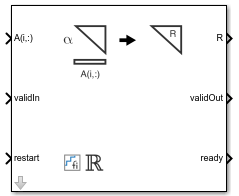# Real Partial-Systolic Q-less QR Decomposition with Forgetting Factor

Q-less QR decomposition for real-valued matrices with infinite number of rows

• Library:
• Fixed-Point Designer HDL Support / Matrices and Linear Algebra / Matrix Factorizations

•## Description

The Real Partial-Systolic Q-less QR Decomposition with Forgetting Factor block uses QR decomposition to compute the economy size upper-triangular R factor of the QR decomposition A = QR, without computing Q. A is an infinitely tall real-valued matrix representing streaming data.

The solution to A'Ax = B is x = R\R'\b.

## Ports

### Input

expand all

Rows of real matrix A, specified as a vector. A is an infinitely tall matrix of streaming data. If A uses a fixed-point data type, A must be signed and use binary-point scaling. Slope-bias representation is not supported for fixed-point data types.

Data Types: `single` | `double` | `fixed point`

Whether inputs are valid, specified as a Boolean scalar. This control signal indicates when the data from the A(i,:) input port is valid. When this value is 1 (`true`) and the value of ready is 1 (`true`), the block captures the values at the A(i,:) input port. When this value is 0 (`false`), the block ignores the input samples.

After sending a `true` validIn signal, there may be some delay before ready is set to `false`. To ensure all data is processed, you must wait until ready is set to `false` before sending another `true` validIn signal.

Data Types: `Boolean`

Whether to clear internal states, specified as a Boolean scalar. When this value is 1 (`true`), the block stops the current calculation and clears all internal states. When this value is 0 (`false`) and the value at validIn is 1 (`true`), the block begins a new subframe.

Data Types: `Boolean`

### Output

expand all

Economy size QR decomposition matrix R multiplied by the `Forgetting factor` parameter, returned as a matrix. R is an upper triangular matrix. The output at R has the same data type as the input at A(i,:).

Data Types: `single` | `double` | `fixed point`

Whether the output data is valid, specified as a Boolean scalar. This control signal indicates when the data at output port R is valid. When this value is 1 (`true`), the block has successfully computed the matrix R. When this value is 0 (`false`), the output data is not valid.

Data Types: `Boolean`

Whether the block is ready, returned as a Boolean scalar. This control signal indicates when the block is ready for new input data. When this value is `1` (`true`) and validIn is `1` (`true`), the block accepts input data in the next time step. When this value is `0` (`false`), the block ignores input data in the next time step.

After sending a `true` validIn signal, there may be some delay before ready is set to `false`. To ensure all data is processed, you must wait until ready is set to `false` before sending another `true` validIn signal.

Data Types: `Boolean`

## Parameters

expand all

Number of columns in input matrix A, specified as a positive integer-valued scalar.

#### Programmatic Use

 Block Parameter: `n` Type: character vector Values: positive integer-valued scalar Default: `4`

Forgetting factor applied before each row of the matrix is factored, specified as a real positive scalar. The output is updated as each row of A is input indefinitely.

#### Programmatic Use

 Block Parameter: `forgetting_factor` Type: character vector Values: positive integer-valued scalar Default: `0.99`

expand all

## Extended Capabilities

Introduced in R2020b

## SupportGet trial now